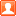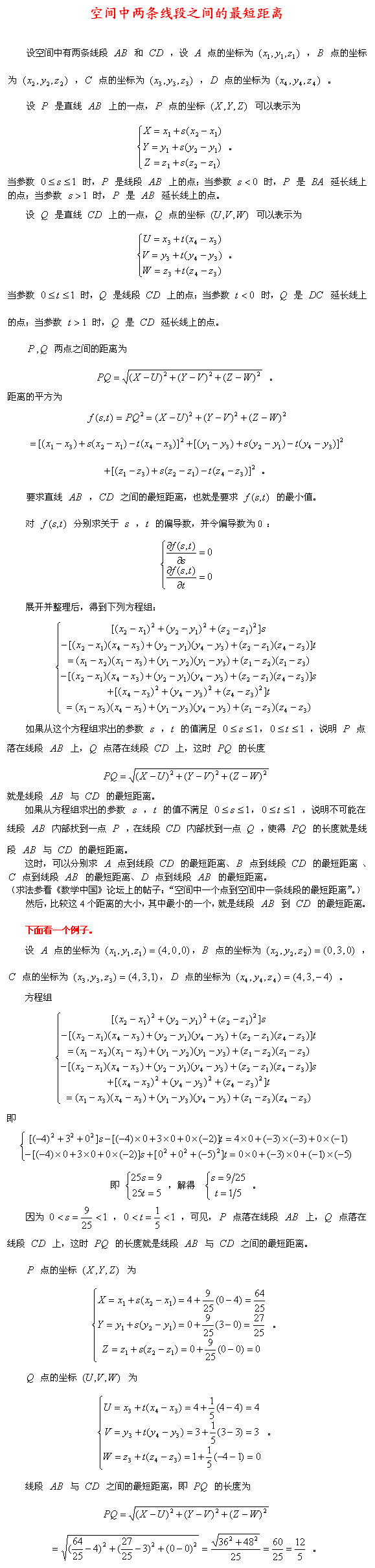##用户名 Email 自动登录 找回密码 密码 注册
 搜索 热搜: 活动 交友 discuz

# 空间中两条线段之间的最短距离发表于 2008-3-13 23:53 | 显示全部楼层 |阅读模式
 本帖最后由 luyuanhong 于 2016-12-14 18:37 编辑191 KB, 下载次数: 8楼主| 发表于 2008-3-16 17:37 | 显示全部楼层

## 空间中两条线段之间的最短距离

 参看《数学中国》论坛上的帖子： “空间中一个点到空间中一条线段的最短距离” http://www.mathchina.com/cgi-bin/topic.cgi?forum=5&topic=3547&show=25 “空间中一个点到空间中一个三角形的最短距离” http://www.mathchina.com/cgi-bin/topic.cgi?forum=5&topic=3564&show=0 “空间中一条线段到空间中一个三角形的最短距离” http://www.mathchina.com/cgi-bin/topic.cgi?forum=5&topic=3565 “[求助]如何求空间两个多面体的最短距离” http://www.mathchina.com/cgi-bin/topic.cgi?forum=5&topic=3511&show=50发表于 2016-12-13 14:35 | 显示全部楼层
 方程组会不会无解？楼主| 发表于 2016-12-14 18:41 | 显示全部楼层
 wudimary111 发表于 2016-12-13 14:35 方程组会不会无解？ 这是一次项系数不等于 0 的一次方程，不可能无解。发表于 2019-11-23 20:08 | 显示全部楼层
 luyuanhong 发表于 2008-3-16 17:37 参看《数学中国》论坛上的帖子： “空间中一个点到空间中一条线段的最短距离” http://www.mathchina.c ... 这几个问题老陆做的不错，ACM竞赛常考的内容。计算几何。发表于 2019-11-24 00:39 | 显示全部楼层
 设空间的二直线的参数方程为 P+tU, Q+tV。 其中 U, V 是直线的方向向量，P，Q 分别是二直线上的点。 若二直线不平行，则所求距离是  |(P-Q)·(U×V)|/|U×V|； 否则所求距离是 |(P-Q)×U)|/|U|发表于 2019-11-24 18:58 | 显示全部楼层
 MATLAB代码：求三维空间任意2直线的最小距离 % Computes the minimum distance between two line segments. % Usage: Input the start and end x,y,z coordinates for two line segments. % p1, p2 are [x,y,z] coordinates of first line segment and p3,p4 are for % second line segment. % Output: scalar minimum distance between the two segments. %  Example: %P1 = [0 0 0];     P2 = [1 0 0]; %  P3 = [0 1 0];     P4 = [1 1 0]; %dist = DistBetween2Segment(P1, P2, P3, P4) %   dist = % %    1 % function [distance varargout] = DistBetween2Segment(p1, p2, p3, p4)     u = p1 - p2;     v = p3 - p4;     w = p2 - p4;         a = dot(u,u);     b = dot(u,v);     c = dot(v,v);     d = dot(u,w);     e = dot(v,w);     D = a*c - b*b;     sD = D;     tD = D;         SMALL_NUM = 0.00000001;         % compute the line parameters of the two closest points     if (D < SMALL_NUM)  % the lines are almost parallel         sN = 0.0;       % force using point P0 on segment S1         sD = 1.0;       % to prevent possible division by 0.0 later         tN = e;         tD = c;     else                % get the closest points on the infinite lines         sN = (b*e - c*d);         tN = (a*e - b*d);         if (sN < 0.0)   % sc < 0 => the s=0 edge is visible                   sN = 0.0;             tN = e;             tD = c;         elseif (sN > sD)% sc > 1 => the s=1 edge is visible             sN = sD;             tN = e + b;             tD = c;         end     end         if (tN < 0.0)            % tc < 0 => the t=0 edge is visible         tN = 0.0;         % recompute sc for this edge         if (-d < 0.0)             sN = 0.0;         elseif (-d > a)             sN = sD;         else             sN = -d;             sD = a;         end     elseif (tN > tD)       % tc > 1 => the t=1 edge is visible         tN = tD;         % recompute sc for this edge         if ((-d + b) < 0.0)             sN = 0;         elseif ((-d + b) > a)             sN = sD;         else             sN = (-d + b);             sD = a;         end     end         % finally do the division to get sc and tc     if(abs(sN) < SMALL_NUM)         sc = 0.0;     else         sc = sN / sD;     end         if(abs(tN) < SMALL_NUM)         tc = 0.0;     else         tc = tN / tD;     end         % get the difference of the two closest points     dP = w + (sc * u) - (tc * v);  % = S1(sc) - S2(tc)     distance = norm(dP);     outV = dP;         varargout(1) = {outV};      % vector connecting the closest points     varargout(2) = {p2+sc*u};   % Closest point on object 1     varargout(3) = {p4+tc*v};   % Closest point on object 2     end发表于 2019-11-24 18:59 | 显示全部楼层
 MATLAB代码：求三维空间任意2直线的最小距离 % Computes the minimum distance between two line segments. % Usage: Input the start and end x,y,z coordinates for two line segments. % p1, p2 are [x,y,z] coordinates of first line segment and p3,p4 are for % second line segment. % Output: scalar minimum distance between the two segments. %  Example: %P1 = [0 0 0];     P2 = [1 0 0]; %  P3 = [0 1 0];     P4 = [1 1 0]; %dist = DistBetween2Segment(P1, P2, P3, P4) %   dist = % %    1 % function [distance varargout] = DistBetween2Segment(p1, p2, p3, p4)     u = p1 - p2;     v = p3 - p4;     w = p2 - p4;         a = dot(u,u);     b = dot(u,v);     c = dot(v,v);     d = dot(u,w);     e = dot(v,w);     D = a*c - b*b;     sD = D;     tD = D;         SMALL_NUM = 0.00000001;         % compute the line parameters of the two closest points     if (D < SMALL_NUM)  % the lines are almost parallel         sN = 0.0;       % force using point P0 on segment S1         sD = 1.0;       % to prevent possible division by 0.0 later         tN = e;         tD = c;     else                % get the closest points on the infinite lines         sN = (b*e - c*d);         tN = (a*e - b*d);         if (sN < 0.0)   % sc < 0 => the s=0 edge is visible                   sN = 0.0;             tN = e;             tD = c;         elseif (sN > sD)% sc > 1 => the s=1 edge is visible             sN = sD;             tN = e + b;             tD = c;         end     end         if (tN < 0.0)            % tc < 0 => the t=0 edge is visible         tN = 0.0;         % recompute sc for this edge         if (-d < 0.0)             sN = 0.0;         elseif (-d > a)             sN = sD;         else             sN = -d;             sD = a;         end     elseif (tN > tD)       % tc > 1 => the t=1 edge is visible         tN = tD;         % recompute sc for this edge         if ((-d + b) < 0.0)             sN = 0;         elseif ((-d + b) > a)             sN = sD;         else             sN = (-d + b);             sD = a;         end     end         % finally do the division to get sc and tc     if(abs(sN) < SMALL_NUM)         sc = 0.0;     else         sc = sN / sD;     end         if(abs(tN) < SMALL_NUM)         tc = 0.0;     else         tc = tN / tD;     end         % get the difference of the two closest points     dP = w + (sc * u) - (tc * v);  % = S1(sc) - S2(tc)     distance = norm(dP);     outV = dP;         varargout(1) = {outV};      % vector connecting the closest points     varargout(2) = {p2+sc*u};   % Closest point on object 1     varargout(3) = {p4+tc*v};   % Closest point on object 2     end发表于 2019-11-27 19:40 | 显示全部楼层
 等于异面直线的距离？楼主| 发表于 2019-11-27 22:48 | 显示全部楼层
 denglongshan 发表于 2019-11-27 19:40 等于异面直线的距离？ 本题要求的，是空间中两条线段之间的最短距离， 并不是空间中两条直线之间的最短距离。 所以本题不能简单地等同于求异面直线之间的距离。

 您需要登录后才可以回帖 登录 | 注册 本版积分规则 回帖并转播 回帖后跳转到最后一页

GMT+8, 2019-12-12 06:44 , Processed in 0.227588 second(s), 20 queries .